# MP Board Class 8th Maths Solutions Chapter 13 Direct and Inverse Proportion Ex 13.1

## MP Board Class 8th Maths Solutions Chapter 13 Direct and Inverse Proportion Ex 13.1

Question 1.
Following are the car parking charges near a railway station upto
4 hours ₹ 60
8 hours ₹ 100
12 hours ₹ 140
24 hours ₹ 180
Check if the parking charges are in direct proportion to the parking time.
Solution: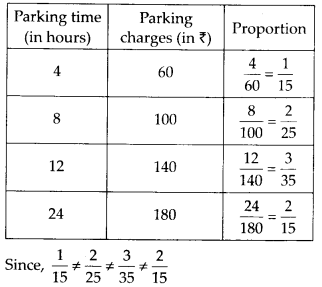∴ The parking timing is not in direct proportion with parking charges.Question 2.
A mixture of paint is prepared by mixing 1 part of red pigments with 8 parts of base. In the following table, find the parts of base that need to be added.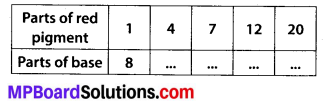Solution: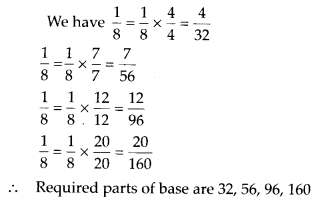Question 3.
In Question 2 above, if 1 part of a red pigment requires 75 mL of base, how much red pigment should we mix with 1800 mL of base?
Solution:
Let x part of red pigment is mixed with 1800 mL of base.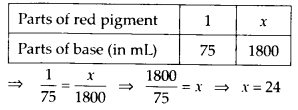Question 4.
A machine in a soft drink factory fills 840 bottles in six hours. How many bottles will it fill in five hours?
Solution:
Let the number of bottles which will be filled in five hours be x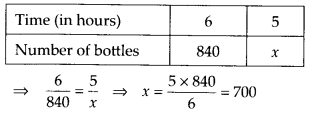Question 5.
A photograph of a bacteria enlarged 50,000 times attains a length of 5 cm as shown in diagram. What is the actual length of the bacteria? If the photograph is enlarged 20,000 times only, what would be its enlarged length?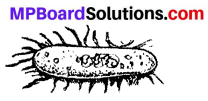Solution:
Let the enlarged length of bacteria be x cm.
∵ 50,000 times enlarged bacteria attains a length of 5 cm.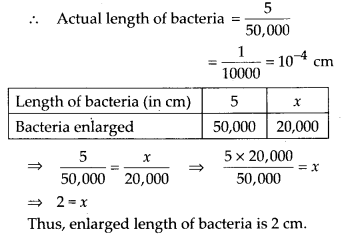Question 6.
In a model of a ship, the mast is 9 cm high, while the mast of the actual ship is 12 m high. If the length of the ship is 28 m, how long is the model ship?
Solution:
Let x m be the length of the model ship when the height of the mast is 9 cm.
Now, according to question,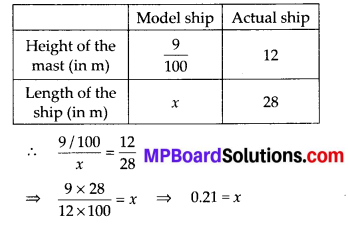Thus, length of the model ship = 0.21 m
= 21 cmQuestion 7.
Suppose 2 kg of sugar contains 9 × 106 crystals. How many sugar crystals are there in
(i) 5 kg of sugar ?
(ii) 1.2 kg of sugar ?
Solution:
Let the crystals contained by 5 kg and 1.2 kg of sugar be x and y respectively.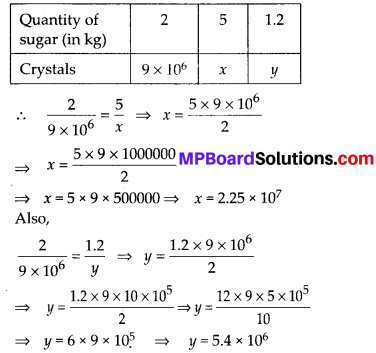Thus, 5 kg and 1.2 kg of sugar contains 2.25 × 107 and 5.4 × 106 crystals respectively.Question 8.
Rashmi has a road map with a scale of 1 cm representing 18 km. She drives on a road for 72 km. What would be her distance covered in the map?
Solution:
Let the distance covered in the map be x cm.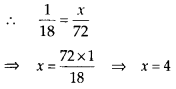Thus, the distance covered by her in the map is 4 cm.Question 9.
A 5 m 60 cm high vertical pole casts a shadow 3 m 20 cm long. Find at the same time
(i) the length of the shadow cast by another pole 10 m 50 cm high
(ii) the height of a pole which casts a shadow 5 m long.
Solution:
Let x be the length of shadow cast by the pole of a height 10 m 50 cm and y be the height of the vertical pole which cast a shadow 5 m long.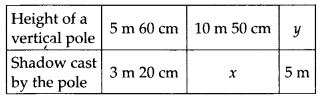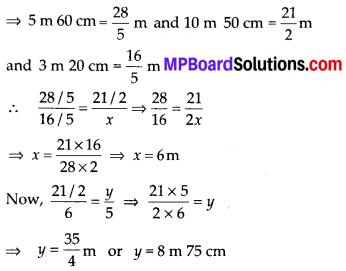Question 10.
A loaded truck travels 14 km in 25 minutes. If the speed remains the same, how far can it travel in 5 hours?
Solution:
Let the distance travelled by truck in 5 hours be x km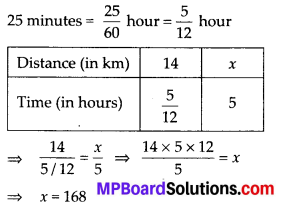∴ Distance travelled by the truck in 5 hours is 168 km.